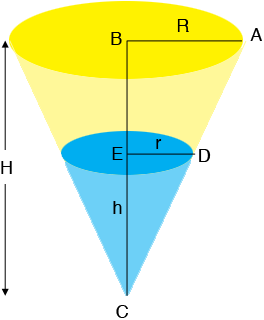SEARCH HOMEMath Central Quandaries & QueriesQuestion from Marcus, a student: Water is running out of a conical funnel at the rate of 1 inch^3/sec. If the radius of the base of the funnel is 4 in. and the altitude is 8 in., find the rate at which the water level is dropping when it is 2 in. from the top.Hi Marcus,

I drew a diagram of the funnel at a time $t$ seconds. My funnel has radius $R$ inches and height $H$ inches. At the time $t$ seconds the radius of the top of the water is $r = r(t)$ inches, the height of the water is $h = h(t)$ inches and the volume $V = V(t)$ in cubic inches is given by

$V(t) = \frac13 \pi\; r^2(t) h(t)$You also know for this problem that $\frac{dV}{dt} = -1$ cubic inches per second and that you want to find $\frac{dh}{dt}$ at a particular time.

The challenge now is to find a relationship between $r(t)$ and $h(t)$ so that you can write the expression for $V(t)$ above in terms of $h(t)$ alone. The fact that I am going to use is that triangles $ABC$ and $DEC$ are similar and hence $\frac{R}{H} = \frac{r}{h}.$ Substitute the values you have for $R$ and $H,$ solve for $r$ and substitute into the equation for $V(t)$ and differentiate both sides of the resulting expression with respect to $t.$ Evaluate this at the time when the water level is 2 inches from the top.

If you have trouble completing this problem write back, tell us what you have done and we will try to help.

PennyMath Central is supported by the University of Regina and The Pacific Institute for the Mathematical Sciences.